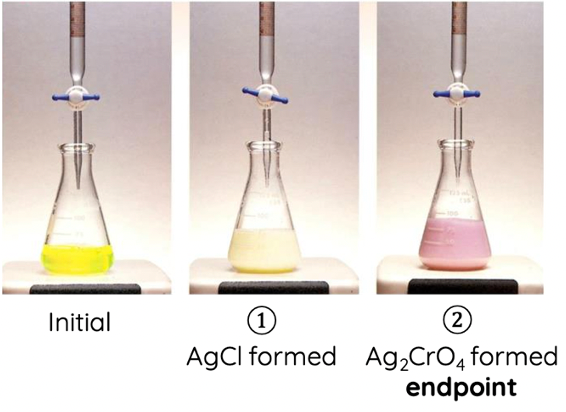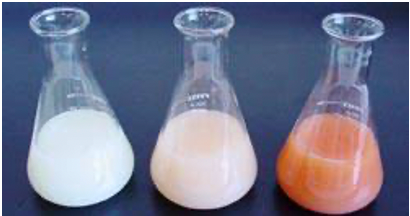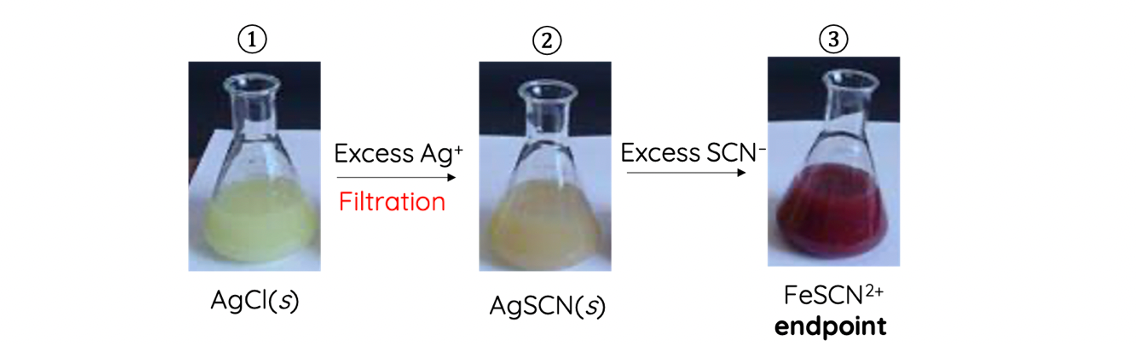# What is Precipitation Titration?

This is part of the HSC Chemistry course under the Module 8 Section 1: Analysis of Inorganic Compounds.

### Precipitation Titration: Mohr's and Volhard's Method

This video explores what precipitation titration is, specifically, Mohr's and Volhard's method of precipitation titration.

## What is Precipitation Titration?

• Precipitation titration is a titration method in which a precipitate is formed. It is a quantitative analytic technique that determines the amount of the analyte by measuring volume.

### Mohr's Method of Precipitation Titration

• Mohr’s method uses AgNO3 as the titrant (burette) and a small quantity of potassium chromate (K2CrO4) as the indicator to determine the concentration of chloride in a given sample.
• For Mohn's method, the titrant is titrated against a supply of chloride ions such that silver chloride and silver chromate precipitate
• Since silver chromate has a much lower Ksp value in theory it should precipitate first

$$K_{sp}(AgCl) = 1.8 \times 10^{-10}$$

$$K_{sp} (Ag_2Cr_2O_4) = 1.2 \times 10^{-12}$$

• However, Mohr’s method uses a small quantity of K2Cr2O4 such that the chloride concentration is much higher than that of chromate. This causes AgCl to preferentially precipitate.
• Silver nitrate is titrated against chloride ions to produce a white precipitate (AgCl).

$$AgNO_3(aq) + Cl^-(aq) \rightarrow AgCl(s) + NO_3^-(aq)$$

• When all chloride ions in a given sample has all been converted to AgCl, additional AgNO3 reacts with yellow K2CrO4(indicator) to produce reddish brown precipitate Ag2CrO4.

$$AgNO_3(aq) + K_2CrO_4(aq) \rightarrow AgCl(s) + NO_3^-(aq)$$• Endpoint is reached when the reddish brown colour remains ‘stable’ – does not disappear with stirring.
• Cl ions are more reactive than CrO42- so the Ag+ ions preferentially react with Cl ions first to form a white precipitate before reacting with CrO42–.Left to right: (1): Cl and CrO42– only, (2): formation of AgCl(s), (3): formation of Ag2CrO4(s)

• Disadvantage of Mohr’s method: can only be carried out between pH of 7 and 10. In acidic environments, chromate (CrO42–) is converted to chromic acid which does not react with excess silver ions. Furthermore, the low concentration of chromate ions in solution is not sufficient to precipitate with silver.

### Volhard's Method of Precipitation Titration

• Volhard’s method uses titrants other than AgNO3g. NH4SCN, and Fe(NO3)3 as the indicator to determine the concentration of chloride in a given sample.
• Known excess quantity of AgNO3 is first mixed with the chloride solution e.g. NaCl. This is placed in the conical flask.

$$AgNO_3(aq) + Cl^-(aq) \rightarrow AgCl(s) + NO_3^-(aq)$$

• Excess unreacted AgNO3 is titrated against NH4SCN (burette) in the presence of Fe(NO3)3 (conical flask).

$$Ag^+(aq) + SCN^-(aq) \rightarrow AgSCN(s)$$• When all of the excess AgNO3 have reacted with the NH4SCN, NH4SCN will start to react with Fe(NO3)3 in the conical flask. This produces a blood red complex iron(III) thiocyanate (FeSCN2+). The red colour will mix with the original yellow appearance to make the solution seem orange (centre flask). When this orange colour remains ‘stable’, endpoint is reached.

$$Fe^{3+}(aq) + SCN^-(aq) \rightarrow FeSCN^{2+}(aq)$$

• Volhard’s method is a variant of back titration. The endpoint indicated by the formation of iron(III) thiocyanate first determines the amount of excess unreacted Ag+. Thus:

number of moles of Cl- =initial mole of AgNO­3 – unreacted AgNO­3

• Volhard’s method is used if the pH of the solution in the conical flask is acidic. If the solution is neutral or basic, Mohr’s method should be used.